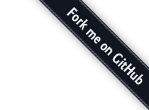## 【背景】

WEB前端研发工程师，在国内是一个朝阳职业，自07-08年正式有这个职业以来，也不过三四年的时间。 这个领域没有学校的正规教育，没有行内成体系的理论指引，几乎所有从事这个职业的人都是靠自己自学成才。 自学成才，一条艰辛的坎坷路，我也是这样一路走来。 从2002年开始接触WEB前端研发至今已然有了9个年头，如今再回首，期间的走了很多弯路。 推已及人，如果能让那些后来者少走些弯路，辛甚辛甚！

## 一.【入门】

``````var str="www.baidu.com/?page";
str=str.replace('?page',"");
str=str.substring(0,str.indexOf("/"));
``````

``````// 计算系统当前是星期几
var str = "";
var week = new Date().getDay();
if (week == 0) {
str = "今天是星期日";
} else if (week == 1) {
str = "今天是星期一";
} else if (week == 2) {
str = "今天是星期二";
} else if (week == 3) {
str = "今天是星期三";
} else if (week == 4) {
str = "今天是星期四";
} else if (week == 5) {
str = "今天是星期五";
} else if (week == 6) {
str = "今天是星期六";
}

// 或者更好一些
var str1 = "今天是星期";
var week = new Date().getDay();
switch (week) {
case 0 :
str1 += "日";
break;
case 1 :
str1 += "一";
break;
case 2 :
str1 += "二";
break;
case 3 :
str1 += "三";
break;
case 4 :
str1 += "四";
break;
case 5 :
str1 += "五";
break;
case 6 :
str1 += "六";
break;
}
``````

”入门”阶段是每个程序员的必经之路，只要“入门”，你就上路了。 所谓“师傅领进门，修行靠个人”，有了这个“入门”的基础，自己就可以摸索着前进了。

【进阶之路】

## 二.【登堂】

``````var str="www.baidu.com/?page";
str=str.replace(/?page/,"");
``````

``````var a = new Array("日", "一", "二", "三", "四", "五", "六");
var week = new Date().getDay();
var str = "今天是星期"+ a[week];
``````

【进阶之路】

## 三.【入室】

``````var str="www.baidu.com/?page";
// 1、字符串剪裁
str.substring(0, str.indexOf("?page"));
// 2、正则表达式
str.replace(/?page/, "");
// 3、字符串分拆、合并
str.split("?page").join("");
``````

“入室”阶段，程序员应该能够肯定的回答：对于这个需求而言，我的代码就是最优秀的代码

``````// 计算系统当前是星期几
var str = "今天是星期" + "日一二三四五六".charAt(new Date().getDay());
``````

• 切勿以为自己能写一手漂亮的代码而自满；
• 切莫以为别人“尊称”你一声“大侠”你就以 “大侠”自居；
• 切莫以为自己积累了一些得意的代码就成了框架式开发。

## 【进阶之路】

• 代码性能的差异、
• 运行平台（浏览器）的差异、
• 需求的隐性扩展、
• 代码的向后兼容等等。

## 四.【入微】

``````var str = "http://www.xxx.com/?pn=0"; // 删除指定字符 pn=0

// 我将这个字符串里所可能想到的各种情况都列举出来
var a = [
"http://www.xxx.com/VMpn=?pn=0"// pn= 可能出现在 ? 前
, "http://www.xxx.com/VMpn=?pn="// URL里允许pn 值为空
, "http://www.xxx.com/VMpn=?pn=0&a=1"// URL 里可有多个字段
, "http://www.xxx.com/VMpn=?a=1&pn=0"// 可能排在最后
, "http://www.xxx.com/VMpn=?a=1&pn=0&pn=1"// 可能有多个 pn 字段
, "http://www.xxx.com/VMpn=?a=1&pn=0&b=2"// 可能在中间
, "http://www.xxx.com/VMpn=?a=1&pn=0&pn=1&b=1" // 可能在中间成组
, "http://www.xxx.com/VMpn=?a=1&pn=0&b=1&pn=1" // 可能零星分布
];

/* 需求的不言之秘：
? 若出现在字符串最尾则要去之
? & 两个符号不可重叠
*/
var reg = /((\?)(pn=[^&]*&)+(?!pn=))|(((\?|&)pn=[^&]*)+\$)|(&pn=[^&]*)/g;

for (var i = 0; i < a.length; i++) {
alert(a[i] + "\n" + a[i].replace(reg, "\$2"));
}
``````

【进阶之路】

## 五.【化蝶】

``````/**
* 在拼接正则表达式字符串时，消除原字符串中特殊字符对正则表达式的干扰
* @author:meizz
* @version: 2010/12/16
* @param {String} str 被正则表达式字符串保护编码的字符串
* @return {String} 被保护处理过后的字符串
*/
function escapeReg(str) {
return str.replace(new RegExp("([.*+?^=!:\x24{}()|[\\]\/\\\\])", "g"), "\\\x241");
}

/**
* 删除URL字符串中指定的 Query
* @author:meizz
* @version:2010/12/16
* @param {String} url URL字符串
* @param {String} key 被删除的Query名
* @return {String} 被删除指定 query 后的URL字符串
*/

function delUrlQuery(url, key) {
key = escapeReg(key);
var reg = new RegExp("((\\?)("+ key +"=[^&]*&)+(?!"+ key +
"=))|(((\\?|&)"+ key +"=[^&]*)+\$)|(&"+ key +"=[^&]*)", "g");
return url.replace(reg, "\x241")
}

// 应用实例
var str = "http://www.xxx.com/?pn=0"; // 删除指定字符 pn=0
delUrlQuery(str, "pn");
``````

1. 不再是就事论事，头疼医头，而是把一类问题抽象理论化，一招破万招；
2. 有封装的概念，不再是每次从零开始，而是站在半山腰开始爬。

【进阶之路】

## 六.【大侠】

``````// 库文件 /mz/string/escapeReg.js
/**
* 在拼接正则表达式字符串时，消除原字符串中特殊字符对正则表达式的干扰
* @author:meizz
* @version: 2010/12/16
* @param {String} str 被正则表达式字符串保护编码的字符串
* @return {String} 被保护处理过后的字符串
*/
mz.string.escapeReg = function (str) {
return str.replace(new RegExp("([.*+?^=!:\x24{}()|[\\]\/\\\\])", "g"), "\\\x241");
}

// 库文件 /mz/url/delQuery.js
/// include mz.string.escapeReg;
/**
* 删除URL字符串中指定的 Query
* @author:meizz
* @version:2010/12/16
* @param {String} url URL字符串
* @param {String} key 被删除的Query名
* @return {String} 被删除指定 query 后的URL字符串
*/
mz.url.delQuery = function (url, key) {
key = mz.string.escapeReg(key);
var reg = new RegExp("((\\?)("+ key +"=[^&]*&)+(?!"+ key +
"=))|(((\\?|&)"+ key +"=[^&]*)+\$)|(&"+ key +"=[^&]*)", "g");
return url.replace(reg, "\x241")
}

// 应用实例
/// include mz.url.delQuery;
var str = "http://www.xxx.com/?pn=0"; // 删除指定字符 pn=0
mz.url.delQuery(str, "pn");
``````

``````// 库文件 /mz/url/delQuery.js
/// include mz.string.escapeReg;
/**
* 删除URL字符串中指定的 Query
* @author:meizz
* @version:2010/12/16
* @param {String} url URL字符串
* @param {String} key 被删除的Query名
* @return {String} 被删除指定 query 后的URL字符串
*/
String.prototype.delQuery = function ( key) {
key = mz.string.escapeReg(key);
var reg = new RegExp("((\\?)("+ key +"=[^&]*&)+(?!"+ key +
"=))|(((\\?|&)"+ key +"=[^&]*)+\$)|(&"+ key +"=[^&]*)", "g");
return this.replace(reg, "\x241")
}

// 应用实例
/// include mz.url.delQuery;
var str = "http://www.xxx.com/?pn=0"; // 删除指定字符 pn=0
str.delQuery("pn");
``````

【进阶出路】

【进阶出路】

## 八.【飞升】